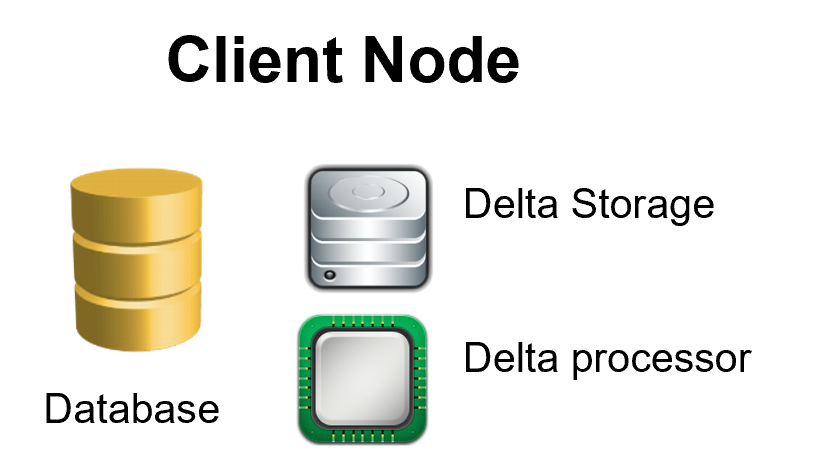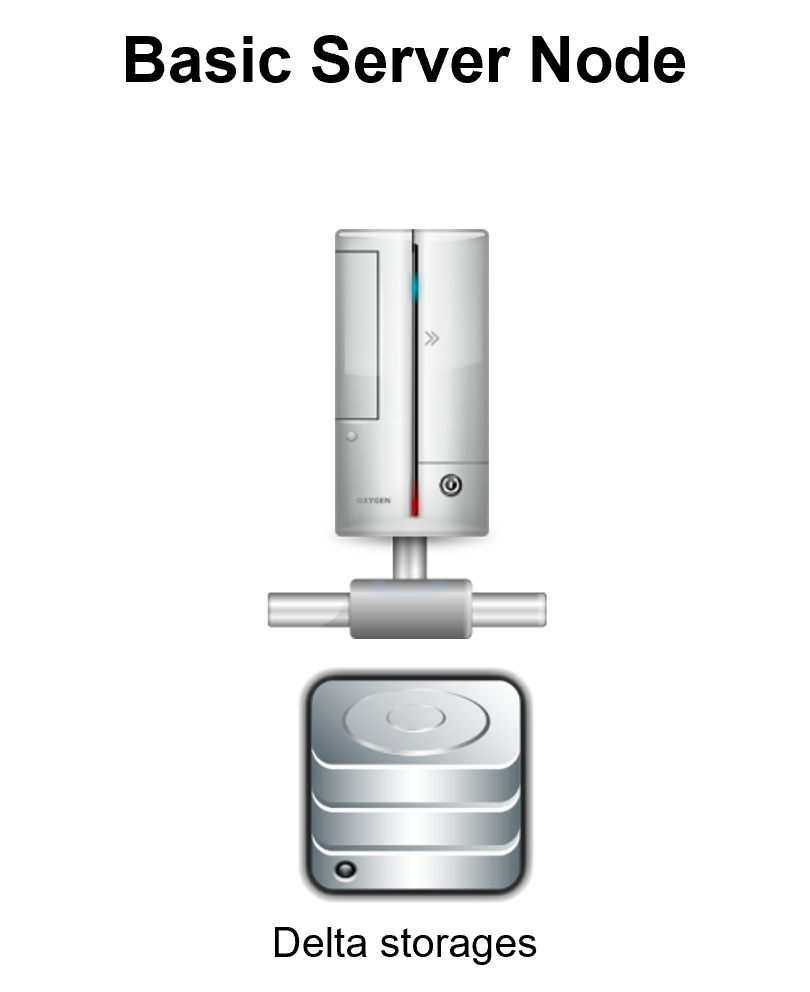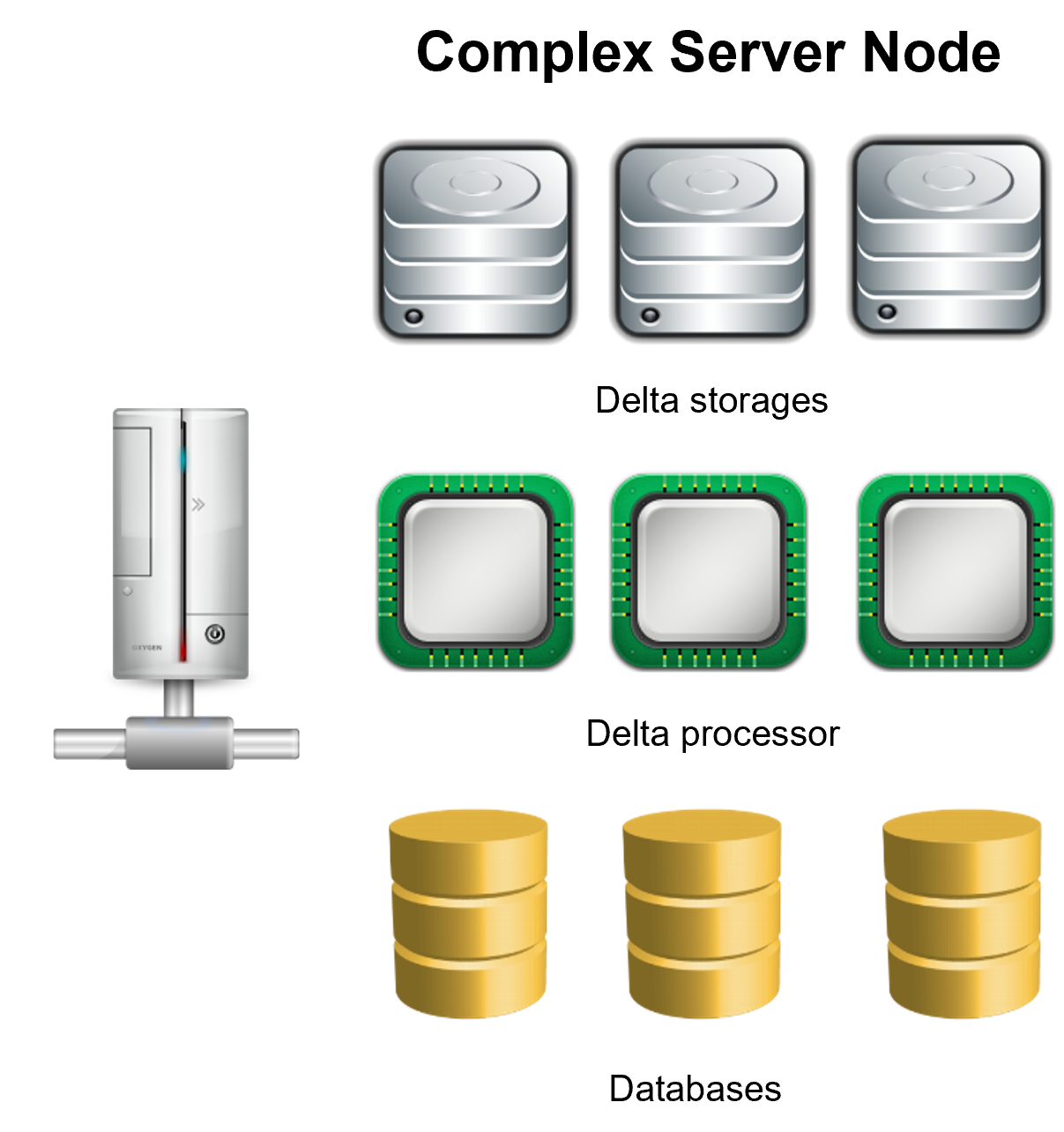Select Page

In the last post, we talked about what are deltas and how by using them we can synchronize data structures.

So, in this post, I will describe the necessary parts needed to implement Delta-based synchronization, let’s start

• Data Object: any database, object, graph, or file system that we are tracking for synchronization
• Delta: a delta is a data difference between the original and the current state of a data object
• Node: is a point of the synchronization network, there are 2 types of nodes
1. Client node: a node that is able to produce and process deltas
2. Server node: a node that is used only to exchange deltas, it can optionally process deltas too.
• Delta Store: storage where you can save deltas so you can later exchange them with other nodes
• Delta Processor: a utility that helps you includes the deltas created in other nodes in your current copy of the data object

Now let’s implement this concept to synchronize a database

Ok so each database that we want to synchronize will be a client node and a client node should be able to produce and store deltas and to process deltas produced by other nodes, so our database node should look like the following diagramThe server node can be as simple as just delta storage exposed in an HTTP API as you can see in the basic server node diagram or use several delta storages and delta processors as show on the complex server node diagramAnd with those diagrams, I finish this post, in the next post we will implement those concepts in C# code